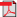# Search Results

1. E. Hyytiä, P. Lassila, L. Nieminen and J. Virtamo, Spatial Node Distribution in the Random Waypoint Mobility Model, COST279, TD(04)029, 2004(bib)
Abstract: The random waypoint model (RWP) is one of the most widely used mobility models in performance analysis of ad hoc networks. We analyze two key properties of the RWP model in a given convex area: the stationary spatial distribution for the location of the node and the mean length of a leg (line between two waypoints). A general expression is given for the spatial node distribution in the form of a one-dimensional integral, giving the density up to a normalization constant. The mean length of a leg can be obtained through the normalization constant or by an alternative expression in the form of a two-dimensional integral. Similar analytical results are also given for a modified version of RWP, where the waypoints are chosen randomly on the perimeter. Additionally, we utilize our analytical results and present several simulation methods that allow an effcient generation of samples from the spatial node distribution. The analytical results are illustrated through numerical examples.